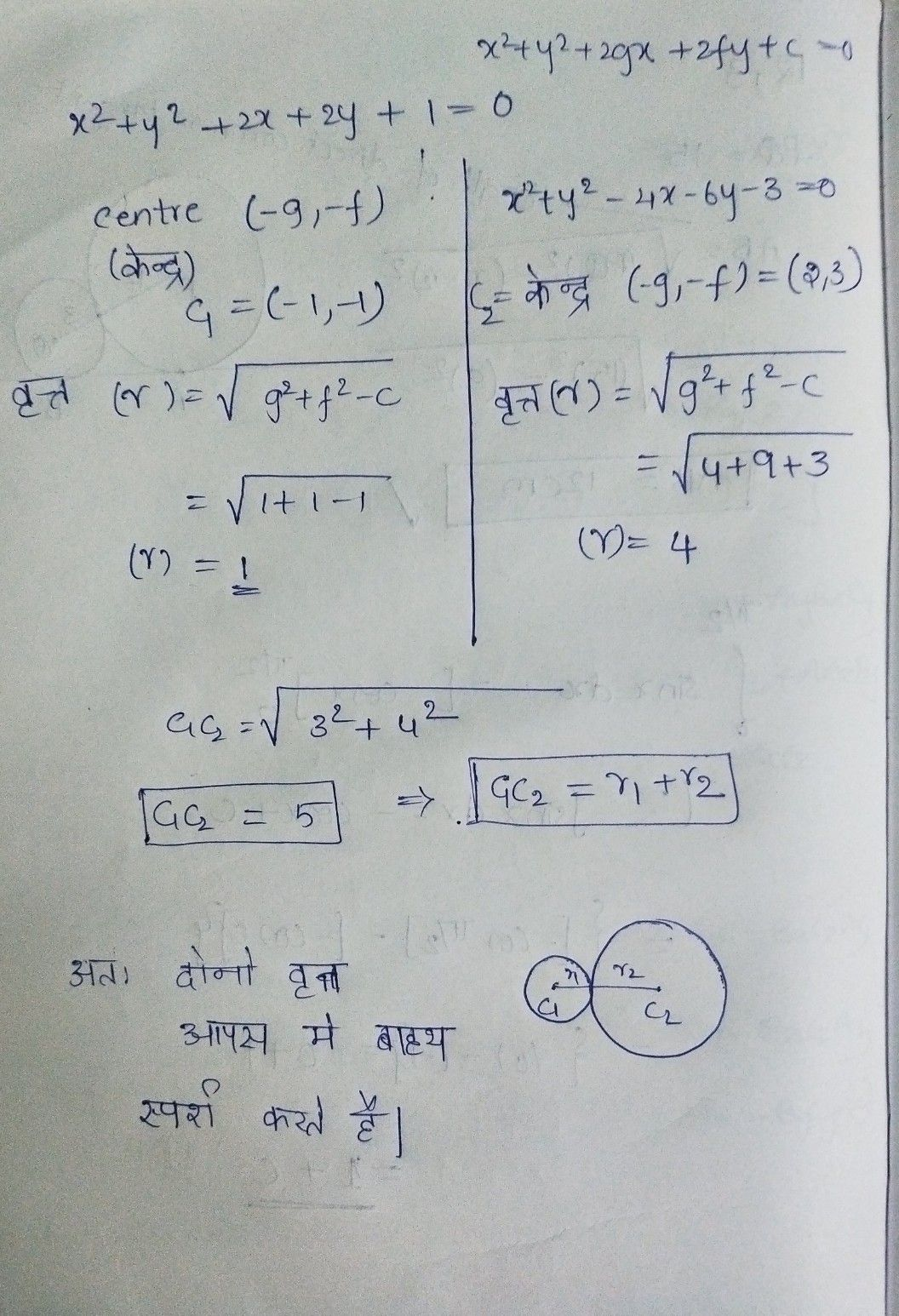Symbol
Problem$7.$ f a f $x^{2}+y^{2}+2x+2y+1=0$ $x^{2}+y^{2}-4x-6y-3=0$
Geometry
SolutionQanda teacher - NIKHIL1